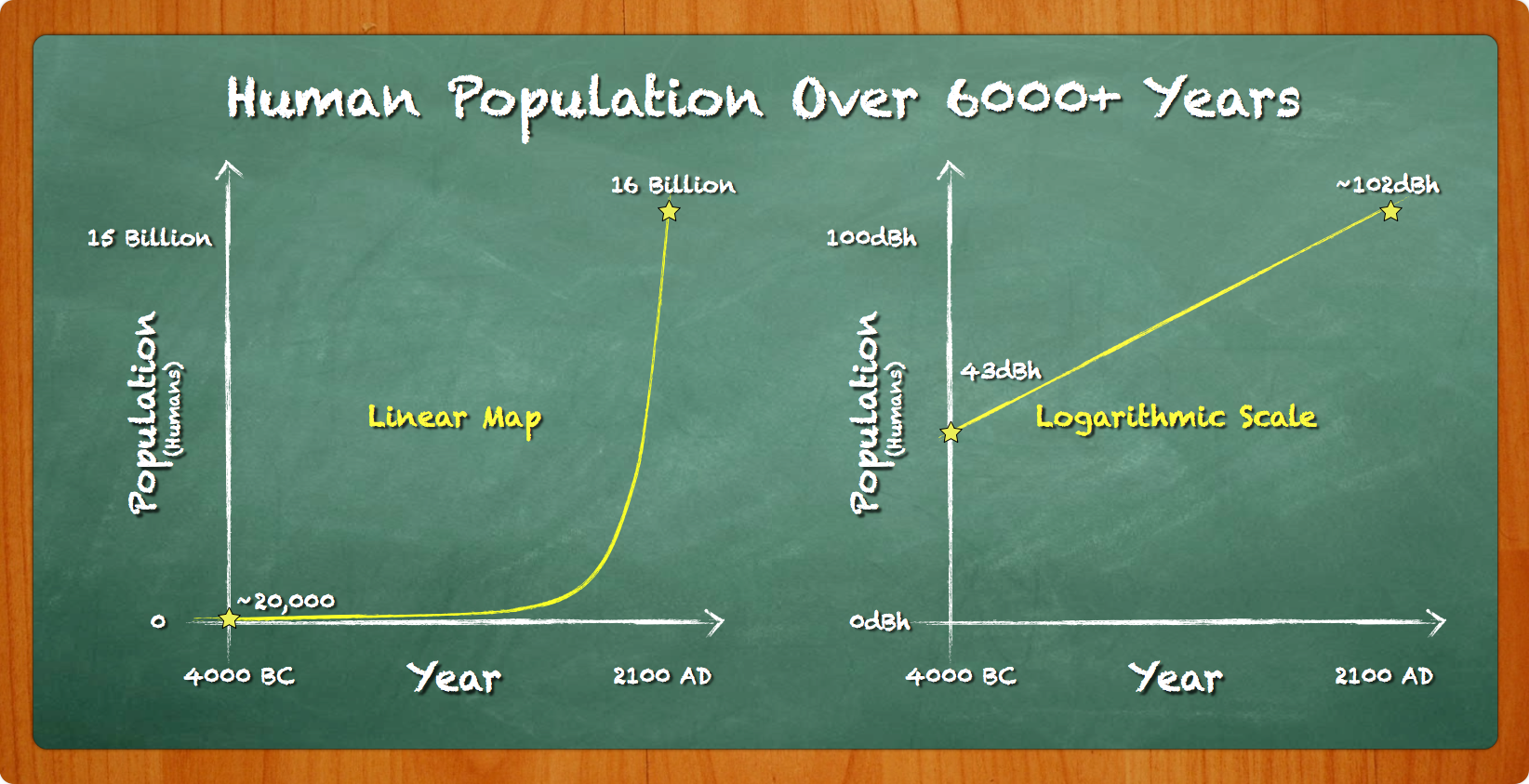UISP
Support# Intro to Networking - Decibels (dB) in Radio Systems

2023-08-08 03:28:11 UTC

# Overview

This article describes how to calculate real-world values from decibels (abstract representations). This is particularly important in the RF world where decibels are used in link budgets. Find this article and other similar ones in the UBWA Training Guide.

# Introduction: What are decibels?

Decibels are a convenient way of expressing very large or very small numbers. They take an exponential curve and make it look like a straight line. By itself, a decibel means nothing. Decibels are simply used when representing something. Anything! Here are just a few ways decibels can represent "real-world" values:

• "dBm", a power ratio. 0 "dBm" references to 1mW
• "dBp", a population ratio. 0 "dBp" references to 1 person
• "dBo", a molecular ratio. 0 "dBo" references to 1 oxygen molecule

Do you notice the pattern? Zero "decibels of anything" = 1 of anything.# How are decibels used?

Decibels represent ratios composed of two sides (the decibel side & the "real-world" side). Whatever operation you perform on the decibel side, you must perform the relative function on the real-world side (and vice-versa). There are rules that must be followed when working with either decibels or "real-world" values:

• The decibel side has two functions: addition & subtraction.
• The real-world side has two functions: multiplication & division.
• Every time decibels are added, you must multiply the real-world figure.
• And every time decibels are subtracted, you must divide the real-world figure.

There is a concept known as "the rule of 3's & 10's" which allows you to arrive at any "real-world" number by adding/subtracting 3 and/or 10 decibels.

• +3dB = x2 real-world number
• -3dB = ÷2 real-world number
• +10dB = x10 real-world number
• -10dB = ÷10 real-world number

So if you're trying to arrive at 30dB of something (maybe 30dBm or 30dBo or 30dBz), you will multiply the real-world figure by 1000! (10dB + 10dB + 10dB = x10 x10 x10).

# Examples

A few examples are provided for practice.

```A radio transmitter is outputting 13dBm. How many milliwatts is this?Where do you start when calculating decibels? When dealing with decibels, always start at ZERO. After all, 0dB = 1 of anything, right?We start at 0dBm. How many milliwatts do we have total?
0dBm = 1mW.
Now we need to get from 0dBm to 13dBm. According to the "rule of 3's & 10's," we can't add "13dBm" (it's not the rule of 13's). But, if we add by 10dBm then add by 3dBm, we have added a total of 13dBm, using just 3's & 10's!
0dBm = 1mW
+3dBm = x2
_____________
3dBm = 2mW
(we're not done yet)
3dBm = 2mW
+10dBm = x10
_____________
13dBm = 20mW
So, 13dBm equals 20mW.```
```Now try arriving at 69dBm—get ready, it's a really big number! Always start at ZERO!
0dBm = 1mW
Now what would be easiest? We could add by 3 all day and finally arrive at 69dBm, or we could add by 10 to get to 69dBm more quickly.
0dBm = 1mW
+10dBm = x10
_____________
10dBm = 10mW
10dBm = 10mW
+10dBm = x10
_____________
20dBm = 100mW
20dBm = 100mW
+10dBm = x10
_____________
30dBm = 1,000mW
30dBm = 1000mW
+10dBm = x10
_____________
40dBm = 10,000mW
40dBm = 10,000mW
+10dBm = x10
_____________
50dBm = 100,000mW
50dBm = 100,000mW
+10dBm = x10
_____________
60dBm = 1,000,000mW (really big number!)
Pause!
We're almost there. Now if we add 10dBm to 60dBm, we'll arrive at 70dBm. And since we can't subtract 1dBm from 70dBm (only 3's & 10's!), we will have to add by 3dBm now:
60dBm = 1,000,000mW
+3dBm = x2
_____________
63dBm = 2,000,000mW
63dBm = 2,000,000mW
+3dBm = x2
_____________
66dBm = 4,000,000mW
66dBm = 4,000,000mW
+3dBm = x2
_____________
69dBm = 8,000,000mW
So, 69dBm equals 8,000,000mW.```

How did we arrive at these answers? In both examples, we started at ZERO decibels and used only 3's & 10's to arrive at our answer. We've proven that it's very easy to arrive at a number that is divisible by 3 or 10. What about numbers like 7dBm? Or 8dBm? Or 17dBm?

```How many milliwatts does a 17dBm transmitter produce? . . .Start at ZERO!
0dBm = 1mW
+10dBm = x10
_____________
10dBm = 10mWPause!
We're still not at 17dBm. But if we add by 10, we'll be at 20dBm. And if we add by 3 twice, we'll arrive at 16dBm. And we can't add by 1! Only 3's & 10's. This is where subtraction becomes important. If we add by 10, we arrive at 20dBm. But, we can subtract 3 from 20, and we arrive at 17dBm! Just remember to divide the "real-world side" whenever you subtract from the decibel side.
10dBm = 10mW
+10dBm = x10
_____________
20dBm = 100mW
20dBm = 100mW
-3dBm = ÷2
_____________
17dBm = 50mW```

So, 17dBm = 50mW. There are times when to arrive at the answer, you'll need to add as well as subtract to arrive at the final answer. Here's another example:

```21dBm = ??? mW? Start at ZERO.
0dBm = 1mW
+10dBm = x10
_____________
10dBm = 10mW
10dBm = 10mW
+10dBm = x10
_____________
20dBm = 100mW
20dBm = 100mW
+10dBm = x10
_____________
30dBm = 1,000mW
30dBm = 1,000mW
-3dBm = ÷2
_____________
27dBm = 500mW
27dBm = 500mW
-3dBm = ÷2
_____________
24dBm = 250mW
24dBm = 250mW
-3dBm = ÷2
_____________
21dBm = 125mW
So, 21dBm = 125mW. ```